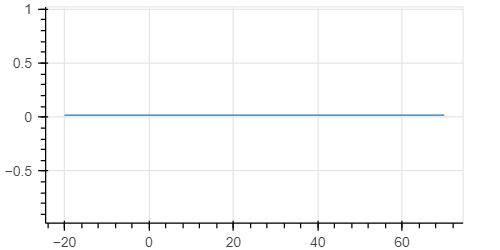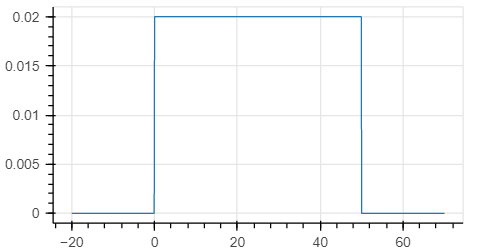# validate_args=True. What does this do?

I noticed that if I run

``````x2_grid = jnp.linspace(-20,70,1000)
sigma= jnp.exp(dist.Uniform(low=0, high=50).log_prob(x2_grid))
``````

I obtain this plotwhile if I run

``````x2_grid = jnp.linspace(-20,70,1000)
sigma= jnp.exp(dist.Uniform(low=0, high=50, validate_args=True).log_prob(x2_grid))
``````

I obtain what I expected, namely:In the documentation it says that this parameter " Whether to enable validation of distribution parameters and arguments to .log_prob method." But it is not clear to me what it means, and why it returns a constant value if I run it without the validation.

with `validate_args=True` the parameters of the distribution and the sample values passed to `log_prob` are checked to see if they satisfy required constraints. for example the `scale` of a `Normal` distribution needs to be positive and a sample value passed to `LogNormal.log_prob` must be positive.

in the case of the uniform distribution the `log_prob` is `log(1/(high - low))` in support and `-inf` out of support. so in the `validate_args=True` case extra checks are done so that you correctly get `-inf` out of support. in the `validate_args=False` case these checks are skipped (for additional speed) under the assumption that you’re on the hook for passing in sample values that are actually in support and results are not guaranteed to be correct (or even not nans) if you pass in sample values that are out of support

1 Like